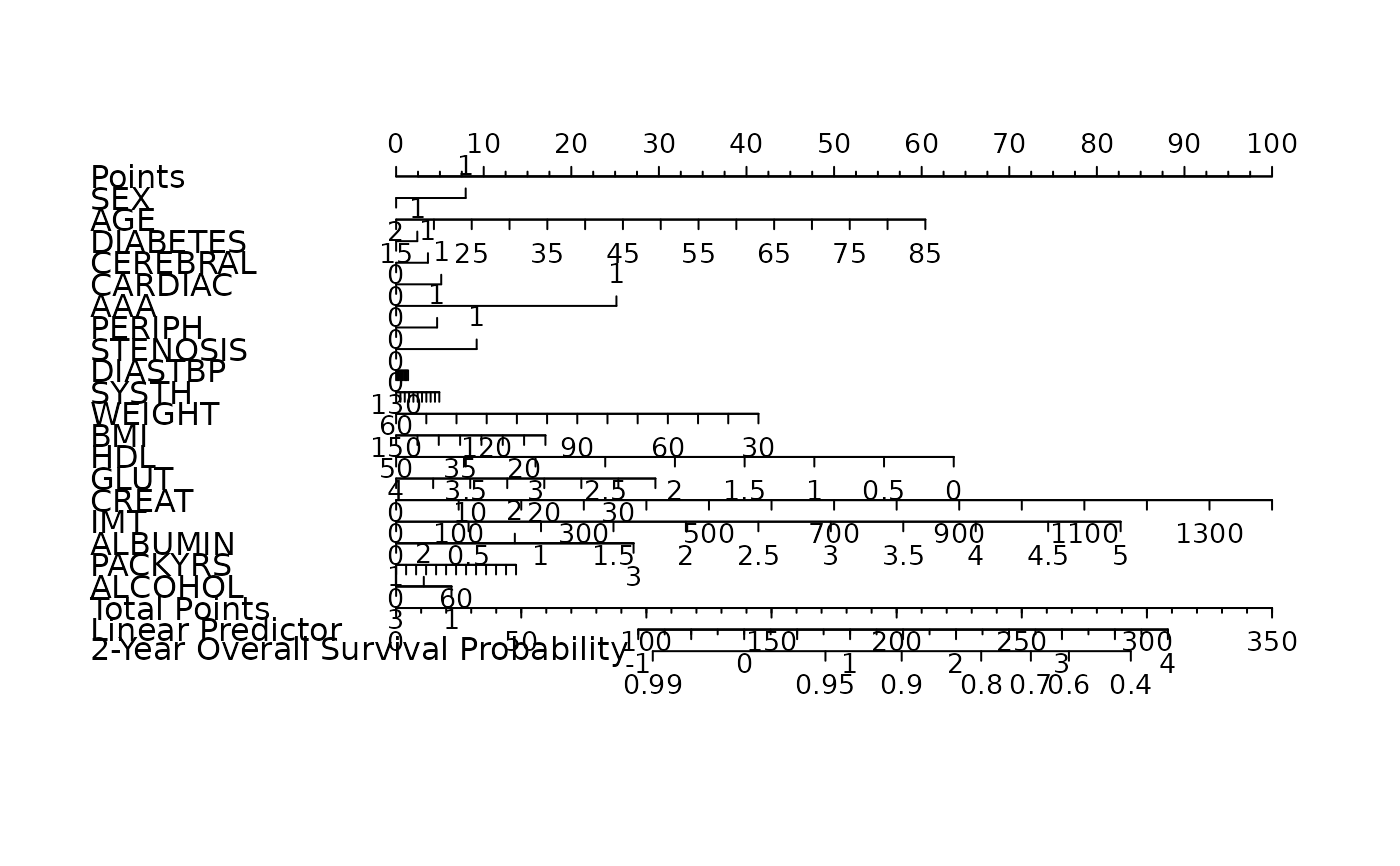Automatic model selection for high-dimensional Cox models with elastic-net penalty, evaluated by penalized partial-likelihood.

fit_enet(x, y, nfolds = 5L, alphas = seq(0.05, 0.95, 0.05),
rule = c("lambda.min", "lambda.1se"), seed = 1001,
parallel = FALSE)

## Arguments

x Data matrix. Response matrix made by Surv. Fold numbers of cross-validation. Alphas to tune in cv.glmnet. Model selection criterion, "lambda.min" or "lambda.1se". See cv.glmnet for details. A random seed for cross-validation fold division. Logical. Enable parallel parameter tuning or not, default is FALSE. To enable parallel tuning, load the doParallel package and run registerDoParallel() with the number of CPU cores before calling this function.

## Examples

data("smart")
x <- as.matrix(smart[, -c(1, 2)])
time <- smart$TEVENT event <- smart$EVENT
y <- survival::Surv(time, event)

# To enable parallel parameter tuning, first run:
# library("doParallel")
# registerDoParallel(detectCores())
# then set fit_enet(..., parallel = TRUE).

fit <- fit_enet(
x, y,
nfolds = 3, alphas = c(0.3, 0.7),
rule = "lambda.1se", seed = 11
)

nom <- as_nomogram(
fit, x, time, event,
pred.at = 365 * 2,
funlabel = "2-Year Overall Survival Probability"
)

plot(nom)